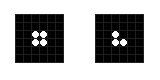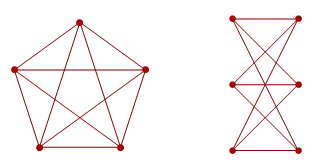# What constitutes an explicit example?

Some proofs of existence provide explicit examples. For instance, a proof of a composite Fermat number is as simple as noting that$2^{32} + 1 = 641 \times 6700417$. On the other hand, some existence proofs are highly non-constructive, and do not provide explicit examples. The proof that the reals can be well ordered cannot be realised as a constructive proof, since the statement is independent of the Zermelo-Fraenkel axioms.

In this post, we’ll consider the borderline between constructive and non-constructive proofs. I have provided four examples, which vary in terms of constructiveness.

### Gardens of Eden

A pattern in Conway’s Game of Life is known as a Garden of Eden if it cannot arise within the successor of another pattern. The proof of the existence of Gardens of Eden relies on the non-injectivity of the rules; two patterns (such as those below) are mapped to the same pattern (namely the one on the left):Now, consider a general$6n \times 6n$ pattern. There are$(2^{36})^{n^2}$ possible patterns of this size, but at most A =$(2^{36} - 1)^{n^2}$ are non-equivalent. The total number of patterns in a$(6n - 2) \times (6n - 2)$ box is B =$2^{(6n - 2)^2}$. As every$k \times k$ box determines a$(k - 2) \times (k - 2)$ box in the following generation, at most A patterns in a box of side length$6n - 2$ are actually constructible.

If we choose n sufficiently large so as to ensure that A < B, then there must exist a Garden of Eden. By writing down the inequality and manipulating it, one can deduce an upper bound of 6859110463024 for the side length of the minimal Garden of Eden. One ‘explicit example’ is the lexicographically first Garden of Eden of this size. A more explicit example is the concatenation of all$2^{6859110463024^2}$ patterns of this size, which must itself be a Garden of Eden by virtue of containing one.

Of course, this argument is pretty pointless as a completely explicit example, which inhabits a$10 \times 10$ box, has already been found.

### Primes with one billion digits

There’s currently a substantial prize offered by the Electronic Frontier Foundation to discover a prime with one billion decimal digits. Due to Bertrand’s postulate, we can be sure that such a prime exists; it is located between$2^{3321928093}$ and$2^{3321928094}$. Hence, we can provide an ‘explicit’ example of a prime with one billion digits, namely the largest prime factor of$(2^{3321928094})!$. However, this seems to be ‘cheating’, and would obviously not win the EFF prize.

### Primitive roots modulo infinitely many primes

An integer n is described as a primitive root modulo p if every number in$\mathbb{Z}_p$ can be expressed as some power of n. For instance, 3 is a primitive root modulo 7, whereas 2 is not. Dr Roger Heath-Brown demonstrated that at most two non-square integers are not primitive roots modulo infinitely many primes. Nevertheless, no numbers have yet been proved to be primitive roots modulo infinitely many primes. So, we know that at least one integer in the set {2, 3, 5} is a primitive root modulo infinitely many primes, and we can take ‘the smallest one satisfying this property’ to be our ‘explicit example’.

### Obstruction sets for toroidal graphs

Wagner’s theorem states that a graph is non-planar if and only if it contains either the complete graph K5 or the bipartite graph K3,3 as a graph minor. The Robertson-Seymour theorem implies that we can find a similar obstruction set for toroidal graphs (graphs that can be drawn on a torus without edges crossing), but we have no idea how large such a set could be.It’s possible, however, to inject the finite sets of graphs into the positive integers. I’ll leave this as an exercise to the reader; it’s not too difficult. Consequently, out of all valid obstruction sets for toroidal graphs, we can choose the one corresponding to the smallest integer. This is still an explicit example in some sense, but even less so than the other examples I’ve provided (there are no size bounds).

If you’ve been affected by any of the issues in this article, please discuss them here in the ‘comments’ section at the foot of the page. In particular, I would like to hear your views on where you draw the line between explicit examples and non-constructive proofs.

This entry was posted in Uncategorized. Bookmark the permalink.

### 0 Responses to What constitutes an explicit example?

1.Joseph Myers says:

Carsten Thomassen’s “A Simpler Proof of the Excluded Minor Theorem for Higher Surfaces” notes how the bounds there and elsewhere give a fully explicit algorithm for finding the excluded minors for a surface. Although the referenced “P. D. Seymour, A bound on the excluded minors for a surface, unpublished manuscript, 1993.” still appears 20 years later to be submitted but not yet published (according to Seymour’s publications list).

2.Johnicholas says:

There’s a distinction between “completely explicit” – meaning “uses the language that the problem itself uses”, and descriptions of one sort or another.

Surely if we are allowing anything less than completely explicit, then we need to fix a language for descriptions of examples, or at least a complexity class for the description language. I’d take an algorithm with a feasible time complexity that outputs a completely explicit example to be a reasonably explicit example – where feasible is the usual blurry “polynomial, with degree and coefficients drawn from the usual small-tailed distribution for these things – mostly 1, 2, 3, 4, essentially never 5.”

3.Kasuha says:

Regarding explicit examples, I’d call it “the more you can put your hands on it, the better”. Or ‘the better you can comprehend it, the better”.

I’d say that there are three levels:
– inconstructible (or impractical) examples. You know they’re correct because they follow your proof, but you cannot verify them by other means. I’d put the first Basilisk or the ‘large’ GoE into that category.
– practical but incomprehensible examples. You know they’re correct because they follow your proof and they can be verified by other means. The later Basilisk is that case, it can be simulated but nobody is able to see it as a whole in full detail.
– practical comprehensible examples. 10×10 GoE or the “bomber” spaceship fall in there.

Of course boundaries between these three are highly subjective and may change over time. Few people would be able to simulate the “small” Basilisk just 10 years ago, for example. And the “large” Basilisk may go up one category in just another 10 years or so.

4.wojowu says:

In 1982 Conway proved GoL has universal computer constructor and thus contains replicator. I consider this proof non-constructive, for pretty obvious reasons. 27 years later you constructed first such device in GoL. It is somewhere stated that tape needed to reconstruct it would have billions of cells and trillions of generations, give or take some to copy tape. But we (at least you) know how to make such tape program. This follows directly from how objects are positioned. If you wished, you really could make one. So I consider your Spartan computer-constructor as sort of explicit example of replicator. Other than that, I wonder when first strictly explicit (i.e. given cell-by-cell) example will be given